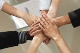# Client Side find control in EditItemTemplate

GridTop achievementsRank 2
Jonathan asked on 27 Jun 2014, 09:09 AM
When grid is in edit mode, I have a need to find controls on client side. My table is rather simple. I have a quantity column and a unit price column, a change in value on either column would update the subtotal column. On either change, I need to get both current control's value (which is easy to retrieve) and the next column control's value, which I have no idea how to retrieve on client side. Can anyone point me to the right direction?

They are all placed under EditItemTemplate.

Thanks

## 2 Answers, 1 is accepted

0
AcceptedPrincy
Top achievementsRank 1
answered on 27 Jun 2014, 10:06 AM
Hi Jonathan,

Please take a look at the below code snippet to set the values to the TextBox in edit mode.

ASPX:
`<``telerik:GridTemplateColumn` `HeaderText``=``"Coulmn1"``>`
`    ``<``EditItemTemplate``>`
`        ``<``telerik:RadNumericTextBox` `ID``=``"rnumtxt1"` `runat``=``"server"` `Value``=``"0"``>`
`        ``</``telerik:RadNumericTextBox``>`
`    ``</``EditItemTemplate``>`
`</``telerik:GridTemplateColumn``>`
`<``telerik:GridTemplateColumn` `HeaderText``=``"Coulmn2"``>`
`    ``<``EditItemTemplate``>`
`        ``<``telerik:RadNumericTextBox` `ID``=``"rnumtxt2"` `runat``=``"server"` `Value``=``"0"``>`
`        ``</``telerik:RadNumericTextBox``>`
`    ``</``EditItemTemplate``>`
`</``telerik:GridTemplateColumn``>`
`<``telerik:GridTemplateColumn` `HeaderText``=``"Total"``>`
`    ``<``EditItemTemplate``>`
`        ``<``telerik:RadNumericTextBox` `ID``=``"rnumtxtTotal"` `runat``=``"server"` `Value``=``"0"``>`
`        ``</``telerik:RadNumericTextBox``>`
`    ``</``EditItemTemplate``>`
`</``telerik:GridTemplateColumn``>`

C#:
`protected` `void` `RadGrid1_ItemCreated(``object` `sender, GridItemEventArgs e)`
`{`
`  ``if` `(e.Item ``is` `GridEditableItem && e.Item.IsInEditMode)`
`  ``{`
`   ``GridEditableItem item = (GridEditableItem)e.Item;`
`   ``RadNumericTextBox rnumtxt1 = item.FindControl(``"rnumtxt1"``) ``as` `RadNumericTextBox;`
`   ``RadNumericTextBox rnumtxt2 = item.FindControl(``"rnumtxt2"``) ``as` `RadNumericTextBox;`
`   ``RadNumericTextBox rnumtxtTotal = item.FindControl(``"rnumtxtTotal"``) ``as` `RadNumericTextBox;`
`   ``rnumtxt1.Attributes.Add(``"onBlur"``, ``"return Onblur1('"` `+ rnumtxt1.ClientID + ``"','"` `+ rnumtxt2.ClientID + ``"','"` `+ rnumtxtTotal.ClientID + ``"')"``);`
`   ``rnumtxt2.Attributes.Add(``"onBlur"``, ``"return Onblur2('"` `+ rnumtxt1.ClientID + ``"','"` `+ rnumtxt2.ClientID + ``"','"` `+ rnumtxtTotal.ClientID + ``"')"``);       `
`  ``} `
`}`

JS:
`<script type=``"text/javascript"``>`
`    ``function` `Onblur1(txt1, txt2, txt3) {`
`        ``debugger;`
`        ``var` `text1 = \$find(txt1); ``// Access 1st textbox`
`        ``var` `text2 = \$find(txt2); ``//Access 2nd textbox`
`        ``var` `text3 = \$find(txt3);`
`        ``var` `total = parseInt(text1.get_textBoxValue()) + parseInt(text2.get_textBoxValue());`
`        ``text3.set_value(total); ``//set value to 3rd textbox`
`    ``}`
`    ``function` `Onblur2(txt1, txt2, txt3) {`
`        ``var` `text1 = \$find(txt1); ``// Access 1st textbox`
`        ``var` `text2 = \$find(txt2); ``//Access 2nd textbox`
`        ``var` `text3 = \$find(txt3);`
`        ``var` `total = parseInt(text1.get_textBoxValue()) + parseInt(text2.get_textBoxValue());`
`        ``text3.set_value(total); ``//set value to 3rd textbox`
`    ``}`
`</script>`

Thanks,
Princy
0Jonathan
Top achievementsRank 2
answered on 13 Aug 2014, 12:18 AM
Thank Princy, it works :)
Tags
GridJonathan
Top achievementsRank 2Rank 1Rank 2